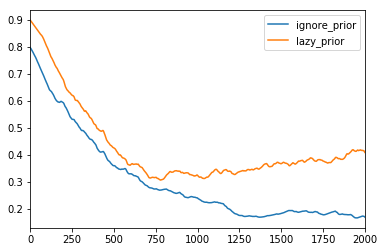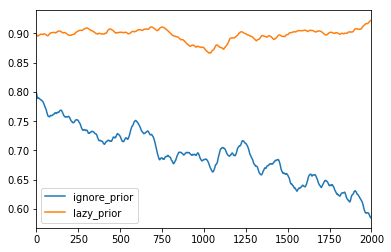Difference between simple ELBO and Trace_ELBO

I’m trying to understand Pyro’s implementation, so I was studying MiniPyro (which has been super useful!). I’ll share a blog post with my experiences soon.

One specific issue I ran into is variational inference with discrete random variables. Specifically, I have a simple model:

``````def sleep_model():
feeling_lazy = sample("feeling_lazy", dist.Bernoulli(0.9))
if feeling_lazy:
ignore_alarm = sample("ignore_alarm", dist.Bernoulli(0.8))
amount_slept = sample("amount_slept",
dist.Normal(8 + 2 * ignore_alarm, 1))
else:
amount_slept = sample("amount_slept", dist.Normal(7, 0.5))
return amount_slept
``````

And I wanted to do a basic analysis on the posterior when observing `amount_slept`.

``````def sleep_guide():
lazy_prior = param("lazy_prior", tensor(0.9), constraint=constraints.interval(0., 1.))
ignore_prior = param("ignore_prior", tensor(0.8), constraint=constraints.interval(0., 1.))
feeling_lazy = sample("feeling_lazy", dist.Bernoulli(lazy_prior))
if feeling_lazy:
sample("ignore_alarm", dist.Bernoulli(ignore_prior))

cond_sleep_model = condition(sleep_model, {"amount_slept": 6.5})
optimizer = Adam({"lr": 0.01, "betas": (0.90, 0.999)})
svi = SVI(cond_sleep_model, sleep_guide, optimizer, loss=Trace_ELBO())

for _ in range(1000):
svi.step()
``````

This has the intended effect of lowering the priors:However, if I use the “simple” ELBO from tutorial:

``````def elbo_loss(model, guide):
guide_trace = trace(guide).get_trace()
model_trace = trace(replay(model, guide_trace)).get_trace()
return -(model_trace.log_prob_sum() - guide_trace.log_prob_sum())

svi = SVI(cond_sleep_model, sleep_guide, optimizer, loss=elbo_loss)
``````

This does not have the intended effect:First, what is the theoretical reason for this issue? MiniPyro says this ELBO only supports “random variables with reparameterized samplers”. Does the Bernoulli distribution not have a reparameterized sampler? If so, are there good resources that explain the issue and why that is?

Second, what is the smallest change I could make to the `elbo_loss` function to work equivalently to `Trace_ELBO` in this specific instance? The actual `Trace_ELBO` source code is fairly complex so I’m trying to implement a more minimal example if possible.

1 Like
``````def elbo_loss_improved(model, guide)
guide_trace = trace(guide).get_trace()
model_trace = trace(replay(model, guide_trace)).get_trace()
model_prob = model_trace.log_prob_sum()
guide_prob = guide_trace.log_prob_sum()
elbo = model_prob - guide_prob
surrogate_elbo = elbo * (guide_prob + 1.) + guide_prob
return -surrogate_elbo
``````

For this specific problem, this adaptation/simplification of the `Trace_ELBO` code seems to work, but I certainly cannot explain why.

you might start by reading this

1 Like